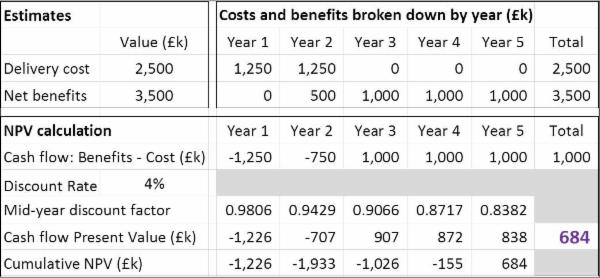# NPV Models

Although NPV can be calculated manually, most NPV forecasts are made by developing a model built using a spreadsheet. The conventional design is to use a table with columns for the different time periods included in the modelled timespan and use rows for the relevant costs and benefits. Calculations can then be aligned under the table.

## Simple Example of an NPV Model

This example has been made simple so as to illustrate the basic mechanics of an NPV model and how they cam be implemented in Excel.

The example project has estimated:

• a delivery cost of £2.5m spread evenly over its first two years and
• net benefits of £3.5m to commence midway through the second year and to be sustained at a rate of £1m per annum until the end of the fifth year.

These should be realistic estimates that include unbiased provision for risk. In this case, they have been made at constant rates i.e. without the effects of inflation.

The figure below illustrates how NPV can be modelled assuming a discount rate of D = 4%. This is a relatively low discount rate, suggesting that the associated market risk is low and also reflecting the fact that inflation has not been factored into the costs and benefits.The present value for the cash flow during each year is calculated by multiplying the cash flow by the relevant discount factor.The project NPV of £684k is in purple font in the cell that calculates the sum of the present values of the cash flows for the five years that comprise the modelled timespan.